## The Basics

Remember a long time ago when you were in Algebra 1 and 2? Yeah, we know, it was a super long time ago. Remember those pesky little problems that were called systems of equations? Usually there were two equations, each with an x and a y variable. Did you really ever know what values you were getting when you solved them, or did you just think they were random x and y values?

This section is all about refreshing our minds on the methods for solving systems of equations: graphically and algebraically. We'll solve systems of equations graphically by finding the intersection of the two lines. We will also solve systems algebraically with the substitution method and elimination method. Prepare to dominate the algebra.

Let's remember what systems of equations are by looking at these two linear equations and trying to find the solution using a little imagination. Don't worry. We won't make you think too hard.

Here is a system of equations that we want to find the solution of:

4x – 2y = 10
2x + 9y = 8

Picture yourself and your best bud each holding the end of a long rope. On a very large coordinate grid (yeah right). You both are situated on a linear equation (4x – 2y = 10). Wait! Do you remember how to plot that? First, get the linear equation in y = mx + b form so you know where the y-intercept and the slope is.

Our rearranged equations are: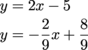Back to our daydreaming. You and your bud are on the y = 2x – 5 equation. Your other two friends are holding that rope that is on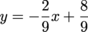.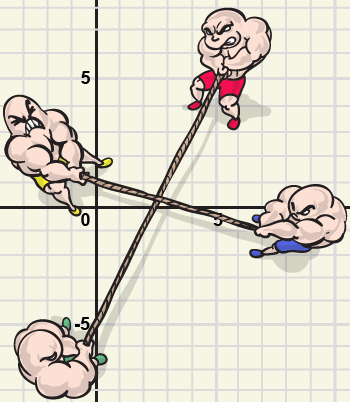The picture above demonstrates this little tug of war, and the solution of these two equations is where these two equations intersect. It's really just as simple as that! The solution of a system of equations is the intersection. Folks, we have a green light. Change your thoughts about what solutions of equations are. They are just where the lines or curves bump into each other.

Have you ever been walking and completely bumped into a stranger? Oops. Sorry. Your intersection was the solution to the system of equations of each of your paths.

Have you ever seen contrails? Those creepy looking trails that airplanes leave at very high altitudes? All conspiracy theories aside, they intersect all the time leaving big X's in the sky. Now we can think of them as a solution.

### Sample Problem

Let's check out that earlier problem using the substitution method. Hopefully this will be a review for you. Remember, we try to get one of the variables in either of the equations isolated.

So our system of equations now look like this:Our goal here is to get you to notice that since y = 2x – 5, we can simply place the 2x – 5 in the y value of the second equation. And this, friends, is the pinnacle to the substitution method. Yes, we believe we have a start to a great math problem. Somebody break out the Nobel Prize.Halfway through that problem, we converted to decimals because sometimes they are easier to work with in problems like this. Now we've got to substitute the x = 2.65 back into either equation. We'll choose the first one since it seems a bit easier, and we like easier.

y = 2x – 5
x = 2.65
y = 2(2.65) – 5 = 0.3

Our solution is (2.65, 0.3) which is where our two lines intersect. Bam!

### Sample Problem

Solve the previous system of equations using elimination:

4x – 2y = 10
2x + 9y = 8

When eliminating variables, our goal is to eventually add the two equations together and knock out one of the variables. When we first look at these above, our first guess would be to multiply row 2 by a -2 because that would cause the x's to be eliminated if you added the equations. Watch and learn, my precious.

First multiply the 2nd equation by -2:

4x – 2y = 10
-2(2x + 9y = 8)

Our equations now look like this:

4x – 2y = 10
-4x – 18y = -16

Now we can add them straight together (which cancels out the x's), solve for y, then plug y back into either equation to find x:Our solution to this system of equations is (2.65, 0.3).

### Sample Problem

Okay, what if the equations weren't always linear. Shut the front door. You've probably noticed that systems of equations were pretty much always two lines. Now we are going to take a look at systems that may not, in fact, be linear. We are going to be on a little learning curve. No problem.

Find the solution to this system of non-linear equations. (Just one of them is non-linear.):

y = 2x2
-3x + 6y = 14

This is going to be a parabola (quadratic) and a line (linear). Notice the little 2 up in the corner of the first equation? You could possibly have none, 1, or 2 intersections. We are going to do this algebraically. The best thing here is to get both equations into the y variable and then set each equation equal to each other. This will eliminate the y variable all together. A little manipulation with the second equation now simplifies our system to this: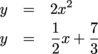Let's set each of the equations equal to each other and solve for x which is going to have a quadratic solution (we'll pull out the quadratic formula for this one):Plug these x values back into one of the original equations, y = 2x2 to find the y values.

y = 2(1.212)2 = 2.939
x = 2(-0.962)2 = 1.852
Solutions: (1.212, 2.939) and (-0.962, 1.852)

### Sample Problem

It's a lot nicer to look at these problems graphically because it simply makes more sense to the human mind. You are a human, aren't you?

Find the solution to this system (from the previous problem) of non-linear equations using technology (graphing calculator like TI-84 or online calculator like desmos.com):

y = 2x2
-3x + 6y = 14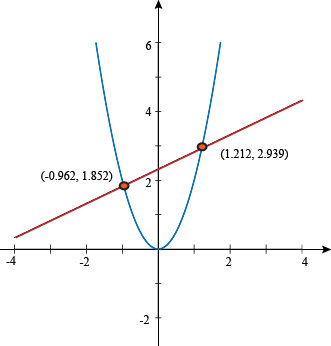Our solution was the same as the previous problem. We have two intersections, so we have two solutions. (-0.962, 1.852) and (1.212, 2.939). Sound familiar?